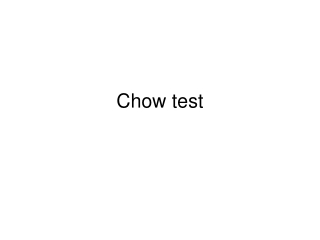Download PresentationChow test

# Chow test

Download Presentation## Chow test

- - - - - - - - - - - - - - - - - - - - - - - - - - - E N D - - - - - - - - - - - - - - - - - - - - - - - - - - -
##### Presentation Transcript

1. Chow test

2. Introduction • Discuss the problems associated with structural breaks in the data. • Examine the Chow test for a structural break. • Assess an example of the use of the Chow test and ways to solve the problem of structural breaks. • Introduce the problem of multicollinearity.

3. Structural Breaks • Structural breaks can occur in time series data or cross sectional data, when there is a sudden change in the relationship being examined. • Examples include sudden policy changes such as a change in government or sudden move in asset prices (1987) or serious international disaster such as a civil war • We then need to decide whether 2 separate regression lines are more efficient than a single regression.

4. Structural Break in 1997

5. Structural Break • In this example a single regression line is not a good fit of the data due to the obvious structural break in 1997. • We need to test if a structural break has occurred in 1997, usually the break is not as obvious as this. • We will use the Chow test, which is a variation of the F-test for a restriction

6. Chow Test (stages in using test) • Run the regression using all the observations, before and after the structural break, collect the RSS • Run 2 separate regressions, one before, RSS(1) and one after, RSS(2) the structural break. • Calculate the test statistic using the following formulae:

7. Chow Test

8. Chow Test • The final stage of the Chow Test is to compare the test statistic with the critical value from the F-Tables. • The null hypothesis in this case is structural stability, if we reject the null hypothesis, it means we have a structural break in the data • We then need to decide how to overcome this break.

9. Chow Test • If there is evidence of a structural break, it may mean we need to split the data into 2 samples and run separate regressions. • Another method to overcome this problem is to use dummy variables (To be covered later in term), the benefit of this approach is that we do not lose any degrees of freedom through a loss of observations.

10. Problems with Chow Test • The test may suggest splitting the data, this may mean fewer degrees of freedom • When should the cut off point be for the test, usually there should be a theoretical basis for this. • There is the potential for structural instability across the whole data range. It is possible to test every observation for a structural break.

11. Multicollinearity • Multicollinearity occurs when two explanatory variables are strongly correlated with each other. • In all multiple regression models there is some degree of collinearity between the explanatory variables, however not enough to cause a serious problem. • However in some cases the collinearity between variables is so high, it affects the regression, producing coefficients with high standard errors.

12. Multicollinearity • It may be that multicollinearity is not a problem if the other conditions are favourable: • High number of observations • Sample variance of explanatory variables is high • Variance of the residual is low

13. Models which can have multicollinearity • Models with large numbers of lags. • Models which use asset returns or interest rates, i.e. 3 month and 10 year interest rates. (This can be overcome by using a term structure of interest rates variable, i.e. one rate minus the other) • Demand models which include different prices of goods.

14. Measuring Multicollinearity • The main way of testing for multicollinearity is to check the t-statistics and R-squared statistic. • If the regression produces a high R-squared statistic (>0.9) but low t-statistics which are not significant, then multicollinearity could be a problem • We could then produce a pair-wise correlation coefficient to determine if the variables are suffering from high levels of multicollinearity. • The problem with this approach is to decide on when the correlation is so large that multicollinearity is present.

15. Remedies for Multicollinearity • Remove one of the variables from the regression which is causing the multicollinearity or alternatively replace it with a variable that is not collinear (This can cause omitted variable bias). • Find data that has more observations. • Transform the variables, i.e. put data into ratio form or take logarithms of the data • Ignore the problem, after all the estimators are still BLUE.

16. Increasing Observations • To overcome multicollinearity, it may be necessary to increase the number of observations by: • Extending data series. • Increasing the frequency of the data, with financial data it is often possible to get daily data. • Pooling the data, it could be that cross section and time series data could be combined.

17. Conclusion • The F-test can be used to test a specific restriction on a model, such as constant returns to scale. • The Chow test is used to determine if the data is structurally stable. • If there is a structural break, we need to split the data or use dummy variables • Multicollinearity occurs when the explanatory variables are closely correlated.